Courses

# Field Effect Transistors - 2

## 15 Questions MCQ Test Topicwise Question Bank for Electrical Engineering | Field Effect Transistors - 2

Description
This mock test of Field Effect Transistors - 2 for Electrical Engineering (EE) helps you for every Electrical Engineering (EE) entrance exam. This contains 15 Multiple Choice Questions for Electrical Engineering (EE) Field Effect Transistors - 2 (mcq) to study with solutions a complete question bank. The solved questions answers in this Field Effect Transistors - 2 quiz give you a good mix of easy questions and tough questions. Electrical Engineering (EE) students definitely take this Field Effect Transistors - 2 exercise for a better result in the exam. You can find other Field Effect Transistors - 2 extra questions, long questions & short questions for Electrical Engineering (EE) on EduRev as well by searching above.
QUESTION: 1

### In the saturation region, the JFET transfer characteristics are

Solution:

The transfer characteristic, giving the relationship between ID and VGS, can be approximated by the parabola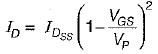QUESTION: 2

### The threshold voltage (VT) of a - MOSFET is defined as

Solution:

The transfer characteristic of an N - MOSFET indicating VT is shown below.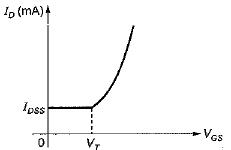QUESTION: 3

### In a JFET, the drain current magnitude decreases as the reverse bias across the gate-channel increases because a larger reverse bias causes

Solution:
QUESTION: 4

The common source JFET amplifier has

Solution:
QUESTION: 5

Assertion (A): In the JFET, the transverse electric field across the reverse biased p-n junction controls the conductivity of the channel.
Reason (R): In enhancement and depletion types of MOSFET, the transverse electric field induced across an insulating layer deposited on the semiconductor material controls the conductivity of the channel.

Solution:
QUESTION: 6

Four important parameters of MOSFET and JFET have comparatively larger or smaller values. In comparison to a JFET, a MOSFET will have which one of the following combinations?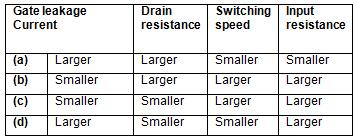Solution: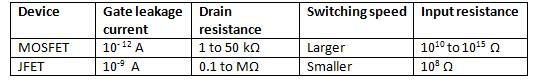QUESTION: 7

Match List-I (Device) with List-II (Applications) and select the correct answer using the codes given below the Lists: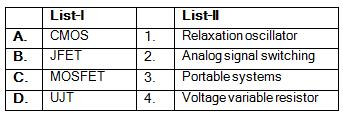Codes: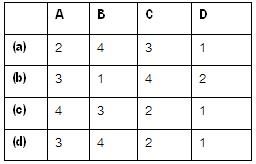Solution:

• Special digital CMOS circuits are available which involve near-zero power dissipation and very low voltage and current requirements. This makes them most suitable for portable systems.
• Since JFET is voltage controlled device, it is used as a voltage variable resistor in operational amplifiers and tone controls.
• Due to zero offset voltage and its symmetrical nature, MOSFET is useful in analog signal switching.
• UJT is used as a relaxation oscillator for generating sawtooth waveforms.

QUESTION: 8

Consider the following statements:
1. In enhancement n-channel MOSFET an induced n-type channel can be produced between the source and drain if VGS is negative.
2. AFET is more sensitive to variations in input voltage in comparison to an ordinary transistor.
3. AFET is characterized by transconductance.
4 UJT has onli one p-n junction and three leads.

Of these statements which are not correct is/are?

Solution:
QUESTION: 9

What are the values of VP and ID for VGS = 0.5 V of an N-channel FET that has IDSS = 10 mA with drain voltage of 5 V and gmo = 5 mA/V?

Solution: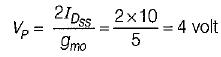and,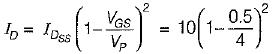= 10 (1 -0.125)2
= 10 x 0.765 = 7.65 mA

QUESTION: 10

An N-channel JFET has IDss = 1mA and VP = -5 V. The value of its maximum transconductance is

Solution: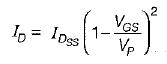and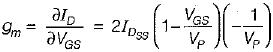∴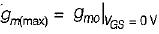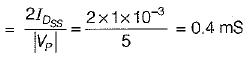QUESTION: 11

N-channel FETs are superior to P-channel FETs, because

Solution:

n-channel FETs are superior to p-chanel FETs because n-channel FETs are faster than p-channel FETs since μn > μP.

QUESTION: 12

In a FET as VGS is changed from zero to increasing reverse bias, the value of gm

Solution: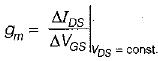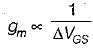QUESTION: 13

A properly biased JFET will act as a

Solution:

Due to high input impedance of JFET, it acts as a voltage controlled current source i.e. IG = 0 A.

QUESTION: 14

A FET is a better chopper than a BJT because it has

Solution:
QUESTION: 15

The variation of drain current with gate-to-source voltage (ID- VGS) characteristic of MOSFET is shown in the figure below.
The MOSFET is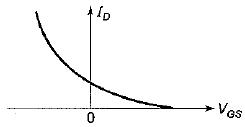Solution: